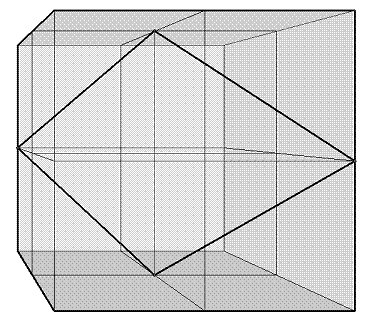#### You may also like### Just Opposite

A and C are the opposite vertices of a square ABCD, and have coordinates (a,b) and (c,d), respectively. What are the coordinates of the vertices B and D? What is the area of the square?A 1 metre cube has one face on the ground and one face against a wall. A 4 metre ladder leans against the wall and just touches the cube. How high is the top of the ladder above the ground?### Hypotenuse Lattice Points

The triangle OMN has vertices on the axes with whole number co-ordinates. How many points with whole number coordinates are there on the hypotenuse MN?

# Something in Common

##### Age 14 to 16Challenge Level

The first part was well explained by Shaun from Nottingham High School as follows:

It is not possible to construct a square of 3 sq. units on a 2-D grid using only integers, because according to Pythagoras the side length must be constructed from the square root of the sum of the squares of two integers.

If the side of the square was tilted and went $x$ along and $y$ up we would need to find $x$ and $y$ so that $x^2 + y^2 =3$

Yet there are no perfect squares which add up to three, hence this is not possible.

Progressing from here to look for a square with an area of 3 in a 3D grid could be tackled using some higher level mathematics involving an operation with vectors called the scalar product (also called the dot product) however it is quite possible to produce a very direct solution using nothing more difficult than Pythagoras and some clear geometric reasoning.

For those of you who wish to explore the vector method, scroll down a bit and you will find a nicely explained solution.
But getting back to the Pythagoras solution . . .
I needed a diagram to help me follow the reasoning so here is a copy of the drawing I made:The only way to get a side length of $\sqrt{3}$ between vertices with whole number coordinates is to go directly across each unit cube.
The path must close in four lengths so there are six choices of direction at the end of the first length (side).
Six, because there are eight cubes around the end point of the first length but one is the route we came along already and one is a continuation in the same direction and that will not lead to a closed path of only four lengths.
Whichever of the six choices we take the quadrilateral closed path will have the same shape in each case.
The quadrilateral I need to examine has four equal sides and so could be a square, but it could also be a rhombus.
It was the diagonals that let me know for sure.
The diagonal length from the start position to the third vertex is $2$ units, but the diagonal length from the second to the fourth position is $\sqrt{8}$
Because the diagonal lengths are not equal the quadrilateral is after all only a rhombus\par I think that exhausts all the possibilities so no solution exists, and there can be no square of side $\sqrt{3}$ whose vertices are all integer values.
A vector approach by Andrei from Tudor Vianu College.

For the second part of the question (3D), a first side of the square is defined by the vector

$\mathbf{a}= \mathbf{i}+\mathbf{j}+\mathbf{k}$

where $\mathbf{i}$, $\mathbf{j}$ and $\mathbf{k}$ are the unit vectors of the axes $Ox$, $Oy$ and $Oz$ respectively.

A second side of the square is represented by the vector $\mathbf{b}$

where

$\mathbf{b}=m\mathbf{i}+n\mathbf{j}+p\mathbf{k}$

Vector $\mathbf{b}$ must have a modulus of $\sqrt{3}$ and form a scalar product with vector $\mathbf{a}$ of zero (the condition for the two directions to be perpendicular)

So the two equations that must be satisfied are :

$m+n+p=0$

and

$m^2+n^2+p^2=3$

There are no integer values for $m$, $n$, and $p$ that satisfy both equations.

So no square with an area of $3$ units can exist on a 3D integer grid.